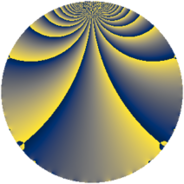Properties

 Label 1859.4.bbLevel $1859$ Weight $4$ Character orbit 1859.bb Rep. character $\chi_{1859}(21,\cdot)$ Character field $\Q(\zeta_{52})$ Dimension $13056$ Sturm bound $728$

Related objects

Defining parameters

 Level: $$N$$ $$=$$ $$1859 = 11 \cdot 13^{2}$$ Weight: $$k$$ $$=$$ $$4$$ Character orbit: $$[\chi]$$ $$=$$ 1859.bb (of order $$52$$ and degree $$24$$) Character conductor: $$\operatorname{cond}(\chi)$$ $$=$$ $$1859$$ Character field: $$\Q(\zeta_{52})$$ Sturm bound: $$728$$

Dimensions

The following table gives the dimensions of various subspaces of $$M_{4}(1859, [\chi])$$.

Total New Old
Modular forms 13152 13152 0
Cusp forms 13056 13056 0
Eisenstein series 96 96 0

Trace form

 $$13056 q - 44 q^{3} - 52 q^{4} - 48 q^{5} - 9764 q^{9} + O(q^{10})$$ $$13056 q - 44 q^{3} - 52 q^{4} - 48 q^{5} - 9764 q^{9} - 40 q^{11} - 52 q^{12} - 252 q^{14} + 28 q^{15} + 17172 q^{16} + 148 q^{20} + 212 q^{22} - 52 q^{25} + 456 q^{26} + 796 q^{27} - 260 q^{31} - 886 q^{33} + 180 q^{34} - 52 q^{36} + 288 q^{37} - 52 q^{38} - 1700 q^{42} + 826 q^{44} + 1096 q^{45} + 228 q^{47} - 2836 q^{48} - 52 q^{49} - 4204 q^{53} + 6530 q^{55} - 52 q^{56} + 1920 q^{58} + 32 q^{59} - 11520 q^{60} - 52 q^{64} + 1932 q^{66} + 10584 q^{67} + 1352 q^{69} + 14720 q^{70} - 1756 q^{71} - 24492 q^{75} - 26 q^{77} + 33460 q^{78} + 3492 q^{80} - 86612 q^{81} + 29588 q^{82} - 4452 q^{86} - 1690 q^{88} + 20324 q^{89} + 5816 q^{91} + 6100 q^{92} + 13684 q^{93} - 9780 q^{97} + 3724 q^{99} + O(q^{100})$$

Decomposition of $$S_{4}^{\mathrm{new}}(1859, [\chi])$$ into newform subspaces

The newforms in this space have not yet been added to the LMFDB.